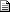# Maths - Clifford / Geometric Algebra - Forum Discussion

On the web page I originally asked:

If a term such as e1^ e1 occurs then this can be replaced by a scalar value, this is because e1^ e1 = 1 or -1 depending on how it is defined. There seems to be a contradiction here because by the anti-commute rule then we should have e1^ e1 = - e1^ e1 which would only be true if e1=0?

This was kindly answered on the forum as follows:

 By: Nobody/Anonymous - nobodygeometric algebra page   2006-02-13 23:32 e1^e1 = -e1^e1    implies that e1^e1=0 not that e1=0    since for scalars a,b    (a e1)^(b e1) = (ab) e1^e1 = -(ab) e1^e1    the a, b cancel, telling us that the above property has to do not with the magnitude of the vectors, but the directions    for instance, think about the product of two vectors    A prod B = |A||B| sin(angle between A,B)    when A,B are in the same *direction*, A prod B = 0

Book Shop - Further reading.

Where I can, I have put links to Amazon for books that are relevant to the subject, click on the appropriate country flag to get more details of the book or to buy it from them.Clifford Algebra to Geometric Calculus: A Unified Language for Mathematics and Physics (Fundamental Theories of Physics). This book is intended for mathematicians and physicists rather than programmers, it is very theoretical. It covers the algebra and calculus of multivectors of any dimension and is not specific to 3D modelling.New Foundations for Classical Mechanics (Fundamental Theories of Physics). This is very good on the geometric interpretation of this algebra. It has lots of insights into the mechanics of solid bodies. I still cant work out if the position, velocity, etc. of solid bodies can be represented by a 3D multivector or if 4 or 5D multivectors are required to represent translation and rotation.

Other Math Books